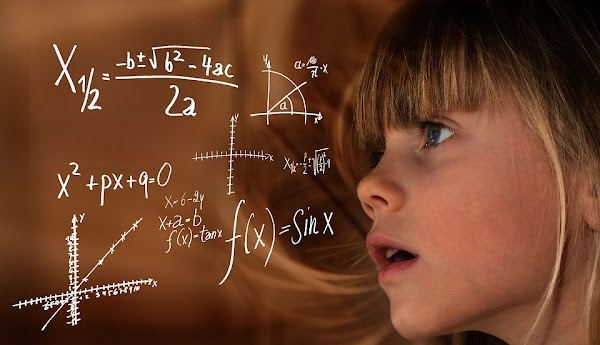## MATHEMATICS NOTES FOR FORM THREE ALL TOPICS

### MATHEMATICS NOTES FOR FORM THREE ALL TOPICSThursday, January 19, 2023
Mathematics (from Ancient Greek μάθημα; máthēma: ‘knowledge, study, learning’) is an area of knowledge that includes such topics as numbers (arithmetic, number theory), formulas and related structures (algebra), shapes and the spaces in which they are contained (geometry), and quantities and their changes (calculus and analysis)

# MATHEMATICS NOTES FOR FORM THREE ALL TOPICS

To view this mathematics notes for form three all topics please hit links bellow

ALL TOPICS

TOPIC 1: RELATIONS

TOPIC 2: FUNCTIONS

TOPIC 3: STATISTICS

TOPIC 4: RATES AND VARIATIONS

TOPIC 5: SEQUENCE AND SERIES

TOPIC 6: CIRCLES

TOPIC 7: THE EARTH AS THE SPHERE

TOPIC 8: ACCOUNTS

Mathematics (from Ancient Greek μάθημα; máthēma: ‘knowledge, study, learning’) is an area of knowledge that includes such topics as numbers (arithmetic, number theory), formulas and related structures (algebra), shapes and the spaces in which they are contained (geometry), and quantities and their changes (calculus and analysis)

Before the Renaissance, mathematics was divided into two main areas: arithmetic — regarding the manipulation of numbers, and geometry — regarding the study of shapes. Some types of pseudoscience, such as numerology and astrology, were not then clearly distinguished from mathematics.

During the Renaissance, two more areas appeared. Mathematical notation led to algebra, which, roughly speaking, consists of the study and the manipulation of formulas. Calculus, consisting of the two subfields infinitesimal calculus and integral calculus, is the study of continuous functions, which model the typically nonlinear relationships between varying quantities (variables). This division into four main areas — arithmetic, geometry, algebra, calculus[verification needed] — endured until the end of the 19th century. Areas such as celestial mechanics and solid mechanics were often then considered as part of mathematics, but now are considered as belonging to physics. Some subjects developed during this period predate mathematics and are divided into such areas as probability theory and combinatorics, which only later became regarded as autonomous areas.

But for more post and free books from our site please make sure you subscribe to our site and if you need a copy of our notes as how it is in our site contact us any time we sell them in low cost in form of PDF or WORD.

Are you a student or a teacher?, are you looking for a good and cheapest study and teaching notes?, Peruzi nasi we offer you with a good and cheapest study and teaching notes in form of PDF and WORD contact us today to get your copy.

SHARE THIS POST WITH FRIEND
MATHEMATICS NOTES FOR FORM THREE ALL TOPICS
4/ 5
Oleh